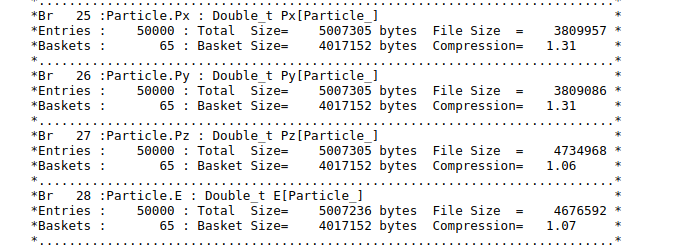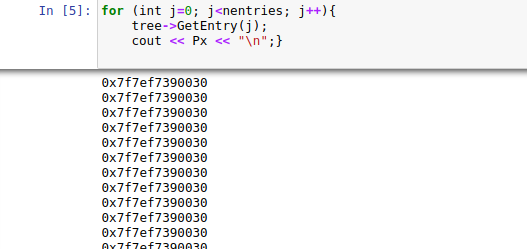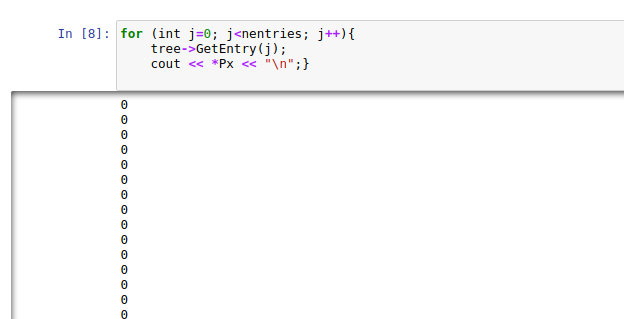# Print Branch with array data

Hi, I want to Print a array in Branch but it Print the address!
//TTree tree= (TTree myfile)->Get(“LHEF”);
TTree *tree = (TTree *) myfile->Get(“LHEF”);

using namespace std;
Double_t Px, Py, Pz;
Int_t PID;
Double_t E;
Long64_t nentries = tree->GetEntries();
for (int j=0; j<nentries; j++){
tree->GetEntry(j);
cout << Px << “\n”;}
` ___`

ROOT Version: 6.18
Platform: Not Provided
Compiler: Not ProvidedHi Sima,

try dereferencing:

``````cout << *Px << "\n";
``````

Hi yus

it didn’t work.
input_line_72:4:13: error: indirection requires pointer operand (‘Long64_t’ (aka ‘long long’) invalid)
cout << *Number << “\n”;}
^~~~~~~

What is `Number`? You did not have it in your example above. Could you maybe show your final code and your ROOT file?I made another code using https://root.cern/doc/master/tree2_8C.html

//TTree tree= (TTree myfile)->Get(“LHEF”);
TTree *tree = (TTree *) myfile->Get(“LHEF”);
Double_t Px;
TBranch *bPx = tree->GetBranch(“Particle.Px”);
TH1F *hdestep = new TH1F(“hdestep”,“destep in Mev”,100,1e-5,3e-5);
Long64_t nentries = tree->GetEntries();
for (Long64_t i=0;i<nentries;i++) {
bPx->GetEntry(i);
//hdestep->Fill(Px);
cout << Px << “\n”;
}

result:

In the tree2_8C.html, the variable they try to histogram is a `static Float_t`. In your example it’s an array of `Double_t`'s.

@yus Can you help me write a correct code?

Sure, but to do that I’ll need your input `ttbar.root`.

https://www.udrop.com/7zw/ttbar.root

Hi Sima,

I figured out how to print these `Particle.Px` values. Not with `TTree::SetBranchAddress()` functionality though, but with `TTreeReader` one. Hope that’s okay:

``````        TFile* myfile = TFile::Open("ttbar.root");

{
if (myReader.GetCurrentEntry() % 10000 == 0)
{